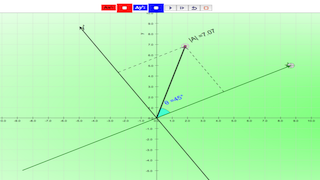## Topics

Measurement of length and time

## Description

A vector is a quantity that has both magnitude and direction. Displacement, velocity, acceleration, and force are the vector quantities. in simpler examples, vectors were simply directed up, down, left or right. In situations in which vectors are directed at angles to the customary x(horizontal)-y(vertical) coordinate axes, a useful mathematical trick will be employed to transform the vector into two parts with each part being directed along the coordinate axes. What if the customary x-y coordinate axes is not (horizontal) and (vertical)? Sometimes, it could be useful to resolve vectors along and perpendicular to a slope, direction of travel than always horizontal and vertical,

## Calculate the components of the vector A in x' and y' direction

1. rotate the x' axis by dragging the GREEN slider to the 2 perpendicular direction to be resolved.
2. change the vector A to be resolved by dragging the BLACK |A| slider and the CYAN angle slider.
3. click reset to generate a new random vector A of length |A| at an angle of theta to the positive x' axis.
4. use the formula Ax' = |A|cos(theta) to find the x' direction component Ax.
5. use the formula Ay' = |A|sin(theta) to find the y' direction component Ay.
6. click reset to random generate a new vector A.
7. test yourself whether you have enough practice calculating Ax' and Ay'.

## Other interesting fun activities

1. this model can be used to calculate any length |A| by keying in the values such as |A| =500 and theta = 53 degrees. Select the checkboxes Ax' and Ay' to see the new answers.
2. when Ax' is selected, the PLAY button animates the effects of changing Ax' alone.
3. when Ay' is selected, the PLAY button animates the effects of changing Ay' alone.

## Sample Learning Goals

(e) state what is meant by scalar and vector (how to resolve) quantities and give common examples of each
(f) calculate vector by means of components in non-horizontal and vertical axes.

## Version:

### Translations

Code Language Translator Run### Software Requirements

SoftwareRequirements

 Android iOS Windows MacOS with best with Chrome Chrome Chrome Chrome support full-screen? Yes. Chrome/Opera No. Firefox/ Samsung Internet Not yet Yes Yes cannot work on some mobile browser that don't understand JavaScript such as..... cannot work on Internet Explorer 9 and below

### CreditsThis email address is being protected from spambots. You need JavaScript enabled to view it.

### Versions:

1. http://www.phy.ntnu.edu.tw/ntnujava/index.php?topic=634.0 sine and cosine by Fu-Kwun Hwang